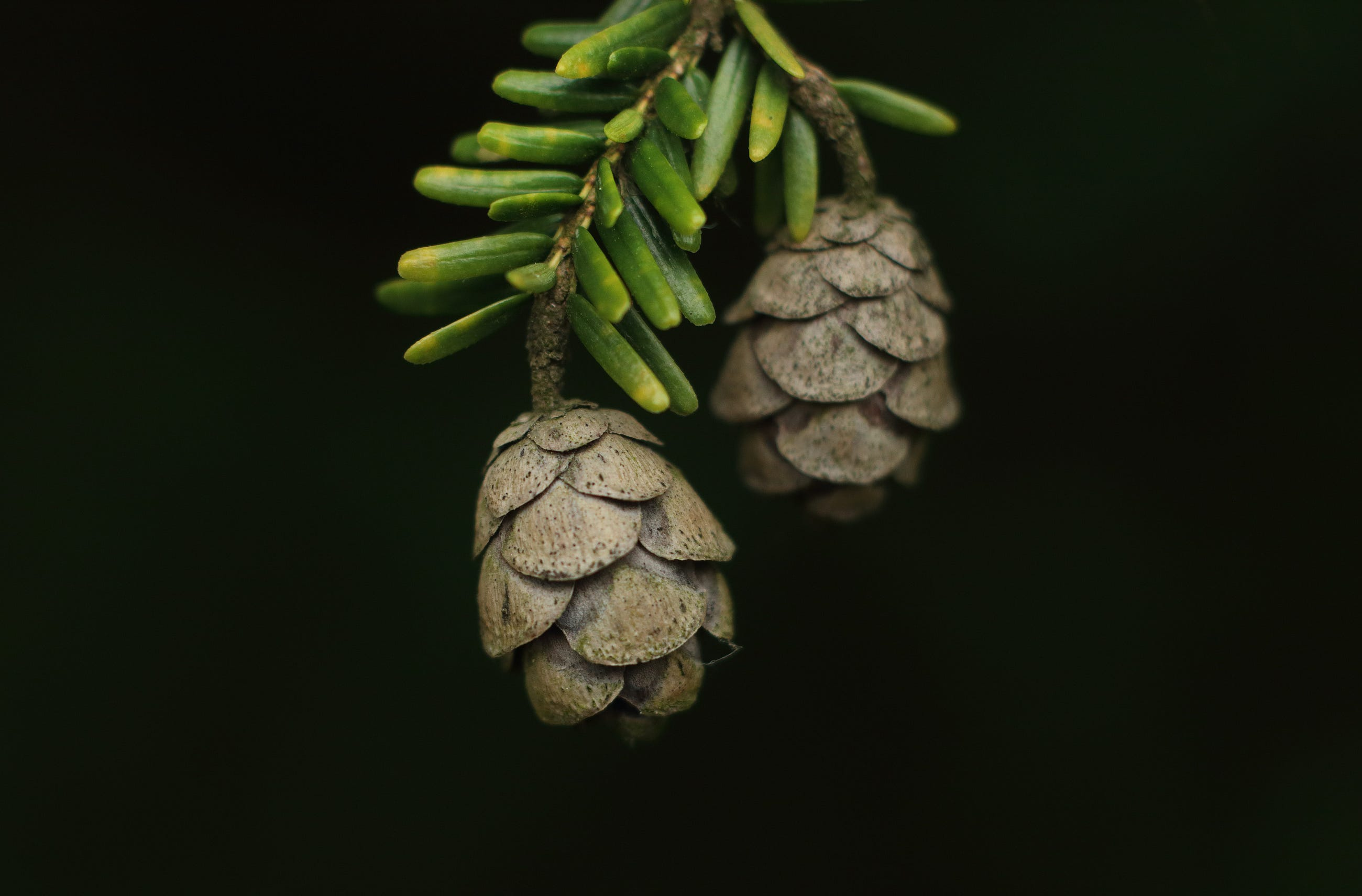# How to Prune Decision Trees to Make the Most Out of Them

Original article was published by Soner Yıldırım on Artificial Intelligence on MediumWe can now start building decision trees with different hyperparameter values. The obvious one is the max_depth which stops tree growth at the specified depth level.

`clf = tree.DecisionTreeClassifier(criterion='gini', max_depth=2)\.fit(X, y)plt.figure(figsize=(16,8))tree.plot_tree(clf, filled=True, fontsize=16)`

The partitions are selected based on Gini impurity and the depth of the tree is 2. Max_depth provides a simple way to control tree growth which may not be enough in more complex cases.

The min_samples_split specifies the minimum number of samples in a node to be further split.

`clf = tree.DecisionTreeClassifier(criterion='gini', min_samples_split=10).fit(X, y)plt.figure(figsize=(20,8))tree.plot_tree(clf, filled=True, fontsize=16)`

I only put a part of the visualization where we see the effect of the current hyperparameter. The node at the right is not further split because there are only 5 samples in it. Without min_samples_split=10, it’d be further split as follows.

Another hyperparameter to control tree growth is min_impurity_decrease which sets a threshold on the impurity decrease to consider a partition. It is a more educated way than the max depth because it takes into account the quality of a partition.

`clf = tree.DecisionTreeClassifier(criterion='gini', min_impurity_decrease=0.1).fit(X, y)plt.figure(figsize=(10, 6))tree.plot_tree(clf, filled=True, fontsize=16)`

All the partitions achieved a decrease of more than 0.1 on the impurity. When setting this value, we should also consider the criterion because Gini impurity and Entropy have different values.

The max_leaf_nodes can also be used to control tree growth. It limits the number of leaf nodes a decision tree can have. The leaf nodes are the nodes at the end of a decision tree.

The tree keeps growing in the best-first fashion until the maximum number of leaf nodes is reached. The best partitions are chosen based on the decrease in impurity.

`clf = tree.DecisionTreeClassifier(criterion='gini', max_leaf_nodes=5).fit(X, y)plt.figure(figsize=(14, 8))tree.plot_tree(clf, filled=True, fontsize=16)`